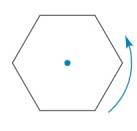Chapter 2.6, Problem 31E### Elementary Geometry for College St...

6th Edition
Daniel C. Alexander + 1 other
ISBN: 9781285195698

#### Solutions

Chapter
Section### Elementary Geometry for College St...

6th Edition
Daniel C. Alexander + 1 other
ISBN: 9781285195698
Textbook Problem
3 views

# A regular hexagon is rotated about acentrally located point (as shown). Howmany rotations are needed to repeat thegiven hexagon, vertex for vertex, if theangle of rotation isa) 30 ° ? b) 60 ° ? c) 90 ° ? d) 240 ° ?

To determine

a)

To find:

The rotation needed to repeat the hexagon vertex-for vertex.

Explanation

Given:

The angle of rotation is 30°.

The given figure is,

Figure (1)

Property:

The hexagon repeats vertex-for vertex if it is rotated for angle of 60° or its multiple angle such as 180°, and 240°

To determine

b)

To find:

The rotation needed to repeat the hexagon vertex-for vertex.

To determine

c)

To find:

The rotation needed to repeat the hexagon vertex-for vertex.

To determine

d)

To find:

The rotation needed to repeat the hexagon vertex-for vertex.

### Still sussing out bartleby?

Check out a sample textbook solution.

See a sample solution

#### The Solution to Your Study Problems

Bartleby provides explanations to thousands of textbook problems written by our experts, many with advanced degrees!

Get Started

#### Rationalize the denominator: x12x.

Applied Calculus for the Managerial, Life, and Social Sciences: A Brief Approach

#### Find y and y. y=lnx1+lnx

Single Variable Calculus: Early Transcendentals

#### The length of a = 4i – j – 2k is: 11 21

Study Guide for Stewart's Multivariable Calculus, 8th